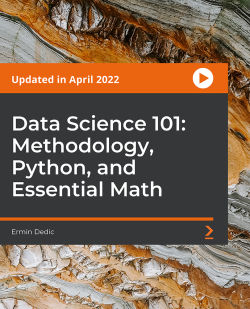# Data Science 101: Methodology, Python, and Essential Math [Video]

By Ermin Dedic
• \$54.99 Video
• \$12.99 Video + Subscription
What do you get with a Packt Subscription?

• Constantly updated with 100+ new titles each month
• Breadth and depth in over 1,000+ technologies

The opening part of Data Science 101 examines some frequently asked questions.

Following that, we will explore data science methodology with a case study. You will see the typical data science steps and techniques utilized by data professionals. Next, you will build a simple chatbot so you can get a clear sense of what is involved.

The next part is an introduction to data science in Python. You will have an opportunity to master Python for data science as each section is followed by an assignment to practice your skills. By the end of the section, you will understand Python fundamentals, decision and looping structures, Python functions, how to work with nested data, and list comprehension. Finally, we will wrap up the two most popular libraries for data science—NumPy and Pandas.

The last part delves into essential math for data science. You will get the hang of linear algebra along with probability and statistics. Our goal for the linear algebra part is to introduce all necessary concepts and intuition for an in-depth understanding of an often-utilized technique for data fitting called least squares. We will spend a lot of time on probability, both classical and Bayesian, as reasoning about problems is a much more difficult aspect than simply running statistics.

By the end of this course, you will understand data science methodology and how to use essential math in your real projects.

All resources are available at https://github.com/PacktPublishing/Data-Science-101-Methodology-Python-and-Essential-Math

Publication date:
April 2022
Publisher
Packt
Duration
14 hours 49 minutes
ISBN
9781803242125